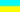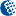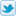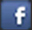Alter.Org.UA<< BackHomeUKDonate### Implementation of CommandLineToArgv for Win32(ANSI and alternative Unicode versions)

I was suprised when found that Win32 API has built-in function for command line parsing. This function is named CommandLineToArgvW(). It builds standard C-style argc/argv pair from command line string. Unfortunately it exists in Unicode version only. Thus, if you want to write simple small programm with command line support but without C-RTL you have two ways:

1. make the programm Unicode-only. In this case Windows 95/98/Me will not be supported
2. write own command line parser

That was the first reason for writing own parser. The second reason is complexity of CommandLineToArgvW() internals. Some time ago I had to write programm for Windows NT Native subsystem. This is the environment of chkdsk, Partition Magic, page defrag, etc. Win32 API is almost unavailable in this mode. The programm used command line parameters. That's why I had to implement CommandLineToArgvW() myself.

After that it was not difficult to implement ANSI version - CommandLineToArgvA(). Find the sources of CommandLineToArgvW() and CommandLineToArgvA() below. To obtain CommandLineToArgvA() make the following replacements in CommandLineToArgvW():

1. WCHAR -> CHAR
2. wcslen -> strlen
3. CommandLineToArgvW -> CommandLineToArgvA
Note: nested quotation marks are not handled , thanks to Igor Levicki for report.

I have met still one application for CommandLineToArgvA() - parsing of parameter string PCHAR args when writing Kernel Debug Extensions.

#### CommandLineToArgvW

```    PWCHAR*
CommandLineToArgvW(
PWCHAR CmdLine,
int* _argc
)
{
PWCHAR* argv;
PWCHAR  _argv;
ULONG   len;
ULONG   argc;
WCHAR   a;
ULONG   i, j;

BOOLEAN  in_QM;
BOOLEAN  in_TEXT;
BOOLEAN  in_SPACE;

len = wcslen(CmdLine);
i = ((len+2)/2)*sizeof(PVOID) + sizeof(PVOID);

argv = (PWCHAR*)GlobalAlloc(GMEM_FIXED,
i + (len+2)*sizeof(WCHAR));

_argv = (PWCHAR)(((PUCHAR)argv)+i);

argc = 0;
argv[argc] = _argv;
in_QM = FALSE;
in_TEXT = FALSE;
in_SPACE = TRUE;
i = 0;
j = 0;

while( a = CmdLine[i] ) {
if(in_QM) {
if(a == '\"') {
in_QM = FALSE;
} else {
_argv[j] = a;
j++;
}
} else {
switch(a) {
case '\"':
in_QM = TRUE;
in_TEXT = TRUE;
if(in_SPACE) {
argv[argc] = _argv+j;
argc++;
}
in_SPACE = FALSE;
break;
case ' ':
case '\t':
case '\n':
case '\r':
if(in_TEXT) {
_argv[j] = '\0';
j++;
}
in_TEXT = FALSE;
in_SPACE = TRUE;
break;
default:
in_TEXT = TRUE;
if(in_SPACE) {
argv[argc] = _argv+j;
argc++;
}
_argv[j] = a;
j++;
in_SPACE = FALSE;
break;
}
}
i++;
}
_argv[j] = '\0';
argv[argc] = NULL;

(*_argc) = argc;
return argv;
}
```

#### CommandLineToArgvA

```    PCHAR*
CommandLineToArgvA(
PCHAR CmdLine,
int* _argc
)
{
PCHAR* argv;
PCHAR  _argv;
ULONG   len;
ULONG   argc;
CHAR   a;
ULONG   i, j;

BOOLEAN  in_QM;
BOOLEAN  in_TEXT;
BOOLEAN  in_SPACE;

len = strlen(CmdLine);
i = ((len+2)/2)*sizeof(PVOID) + sizeof(PVOID);

argv = (PCHAR*)GlobalAlloc(GMEM_FIXED,
i + (len+2)*sizeof(CHAR));

_argv = (PCHAR)(((PUCHAR)argv)+i);

argc = 0;
argv[argc] = _argv;
in_QM = FALSE;
in_TEXT = FALSE;
in_SPACE = TRUE;
i = 0;
j = 0;

while( a = CmdLine[i] ) {
if(in_QM) {
if(a == '\"') {
in_QM = FALSE;
} else {
_argv[j] = a;
j++;
}
} else {
switch(a) {
case '\"':
in_QM = TRUE;
in_TEXT = TRUE;
if(in_SPACE) {
argv[argc] = _argv+j;
argc++;
}
in_SPACE = FALSE;
break;
case ' ':
case '\t':
case '\n':
case '\r':
if(in_TEXT) {
_argv[j] = '\0';
j++;
}
in_TEXT = FALSE;
in_SPACE = TRUE;
break;
default:
in_TEXT = TRUE;
if(in_SPACE) {
argv[argc] = _argv+j;
argc++;
}
_argv[j] = a;
j++;
in_SPACE = FALSE;
break;
}
}
i++;
}
_argv[j] = '\0';
argv[argc] = NULL;

(*_argc) = argc;
return argv;
}
```<< Backdesigned by Alter aka Alexander A. Telyatnikovpowered by Apache+PHP under FBSD© 2002-2023